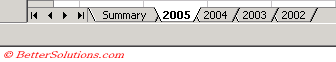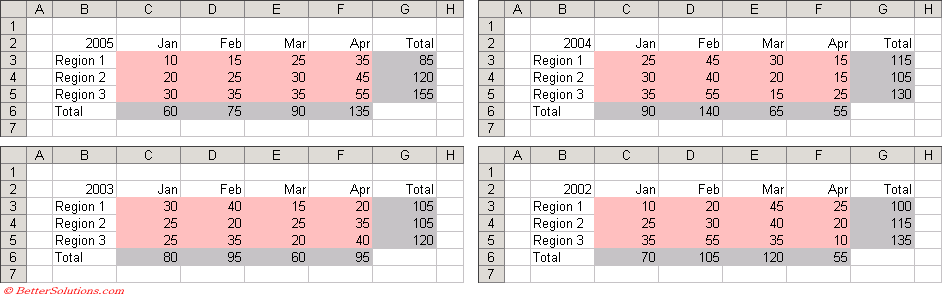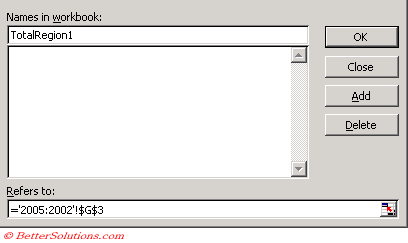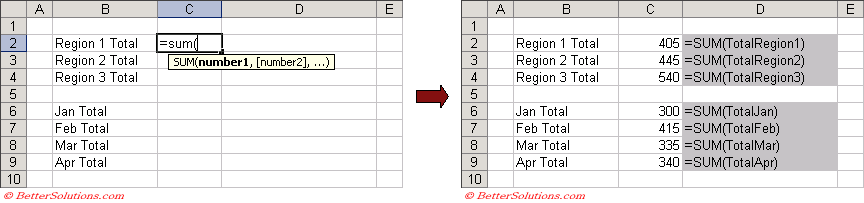# 3D Named Ranges

A 3D named range is a name that spans more than one worksheet.
The selected cell or range of cells must be identical for all the worksheets that are included.
="FirstSheet:LastSheet!RangeReference"
These named ranges must be created using the (Insert > Name > Define) dialog box and not the Name Box.

### What can I use a 3D Named Range for ?

A 3D reference is a reference that refers to the same cell or range on multiple worksheets.
It is possible to use 3D references in your formulas and functions.
The 3D cell reference "=SUM(Sheet1:Sheet4!A2)" can be used to add up the numbers in cell "A2" on 4 different worksheets.
Instead of using this 3D cell reference (Sheet1:Sheet4!A2) you could use a 3D named range instead.

Lets assume that we have a workbook that contains five worksheets and that four of them contain data for specific years.
Four of the worksheets correspond to the sales figures for the years 2005 - 2002 and the first worksheet is intended to be a summary of these four years.On the Summary worksheet we want to be able to quickly return the total for all the Regions and for the months.
It is possible to create 3D named ranges which refer to all four worksheets which will make the formulas in the summary table a lot easier to understand.
Lets assume that each of the four worksheets contains the following table of data.### Defining your 3D Named Range

We are going to define a 3D named range for each of the items we want to total.
The first item in our summary table is the total for Region 1.
Select (Insert > Name > Define) to display the Define Name dialog box.
In the Names box at the top type in the name of your named range, in this case "TotalRegion1"
Click in the Refers to box and press the equal sign (=).
Select the "2005" worksheet tab with the mouse.
Hold down the Shift key and select the "2002" worksheet tab with the mouse.
The formula in the Refers to box should now be ='2005:2002'!'.
Select the cell "G3" on the active worksheet and press "Add" to create the 3D named range.Press OK to close the dialog box.

### Inserting the Formula

Once the 3D named range has been created you can use this named range in your formulas and functions.
It is now extremely easy to obtain the overall total for Region 1.
Create the following table on the Summary worksheet.
In cell "C2" we are going to insert a formula to return the total for Region 1.
We are going to use the 3D named range as the argument for the SUM() function.
It is possible to quickly insert a named range into a formula (or function) by pressing (Insert > Name > Paste) from within the formula (or function).
Type the following and then insert the named range "TotalRegion1".Repeat the above steps for the other six totals to create your summary worksheet.

### Important

If you insert a new worksheet between the worksheets defined in a 3D named range, then the new worksheet will automatically be included.
Deleting either the first worksheet or the last worksheet used in a 3D named range will automatically change the reference to exclude that worksheet.
Deleting any worksheet in the middle of the 3D named range will leave the reference unchanged.
3D Named Ranges do not appear in the Name Box or the (Edit > GoTo) dialog box.
There is no way of selecting the cells which a 3D Named Range refers to.